Question

# Explain (not just the answer) the following: Write an equation for the reaction of each of...

Explain (not just the answer) the following:

Write an equation for the reaction of each of the following acid/base with water (use single or double arrows depending on the strength od the base/acid)

H2SO4

NaClO4

CH2NH2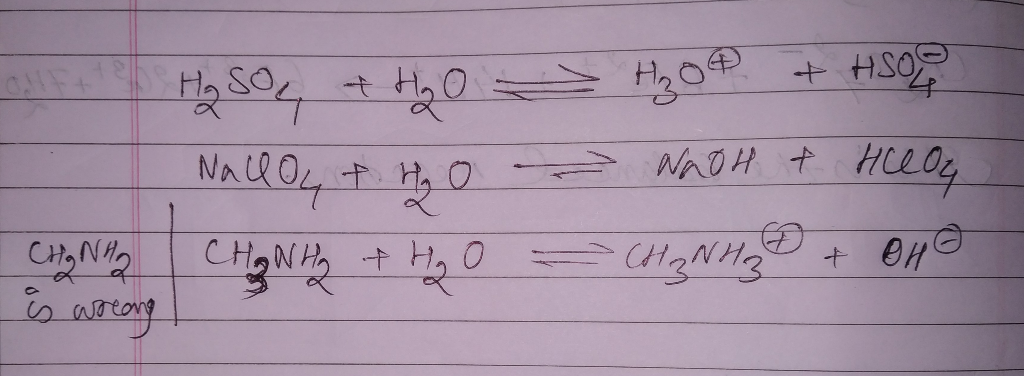#### Earn Coins

Coins can be redeemed for fabulous gifts.

Similar Homework Help Questions
• ### 8. Write an equation for the Bronsted-Lowry acid-base reaction that occurs when the following acids reacts...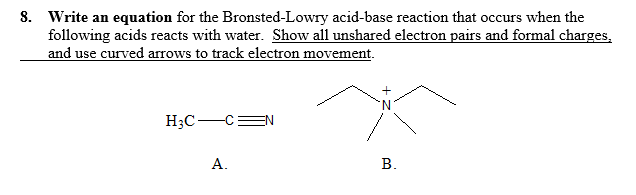8. Write an equation for the Bronsted-Lowry acid-base reaction that occurs when the following acids reacts with water. Show all unshared electron pairs and formal charges, and use curved arrows to track electron movement. H3C-CE A. B.

• ### please answer all parts of this question and explain everything 1. a. Using Pauling's Rules estimate...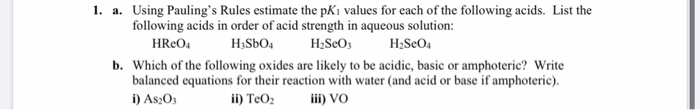please answer all parts of this question and explain everything 1. a. Using Pauling's Rules estimate the pkı values for each of the following acids. List the following acids in order of acid strength in aqueous solution: HRcO4 HzSbO4 H2SO3 H2SO4 b. Which of the following oxides are likely to be acidic, basic or amphoteric? Write balanced equations for their reaction with water and acid or base if amphoteric). i) As2O3 ii) TeO2 ill) VO

• ### Write a net ionic equation to show that nitric acid, HNO3, behaves as an acid in...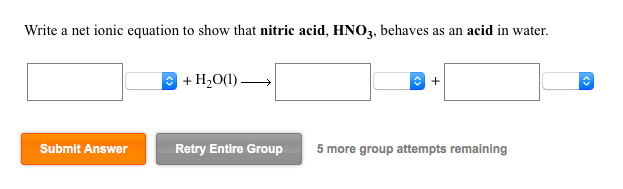Write a net ionic equation to show that nitric acid, HNO3, behaves as an acid in water. + H2o(l) Submit Answer Retry Entire Group 5 more group attempts remaining Use the References to access important values if needed for this question. Acid Conjugate base H,S HS Acid Strength Increases Base Strength Increases HCiO CIO (1) Write a net ionic equation for the reaction between H2S and CIO that shows H2S behavin g as a Bronsted-Lowry acid BL acid BL base...

• ### Complete the equation for the reaction between each Lewis acid-base pair. In each equation, labelwhich starting...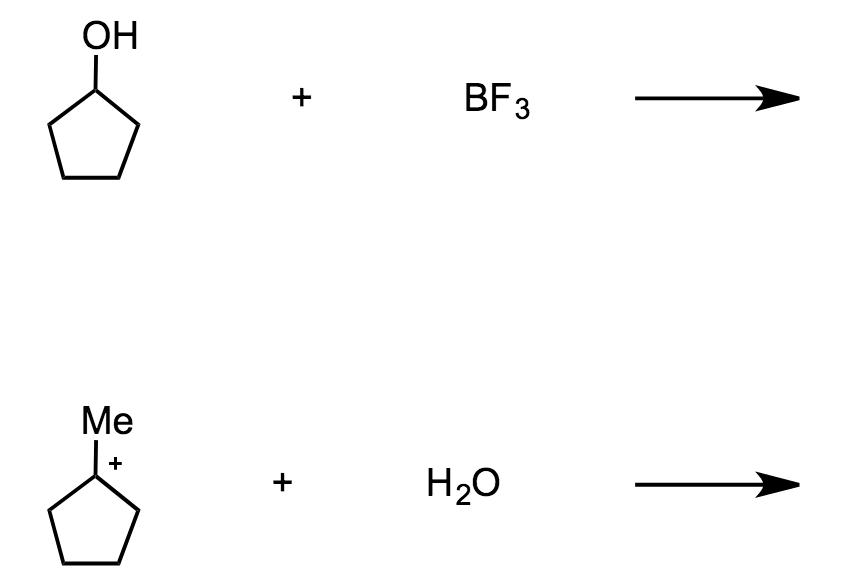Complete the equation for the reaction between each Lewis acid-base pair. In each equation, labelwhich starting material is the Lewis acid and which is the Lewis base. Use curved arrows to show the flow of electrons in each reaction. OH Me BF H2O

• ### Write a balanced chemical equation for each reaction in the experiment. Include the states of all...

Write a balanced chemical equation for each reaction in the experiment. Include the states of all materials. the precipitation reaction between copper(II) nitrate and sodium hydroxide making a precipitate of copper(II) hydroxide and aqueous sodium nitrate the single displacement and oxidation-reduction reaction between excess aqueous hydrochloric acid and aluminum metal to make aqueous aluminum chloride and hydrogen gas the single displacement and oxidation-reduction reaction between aqueous copper(II) chloride and aluminum metal to make aqueous aluminum chloride and metallic copper the...

• ### Indicate what type, or types, of reaction each of the following represents. (Select all that apply.)...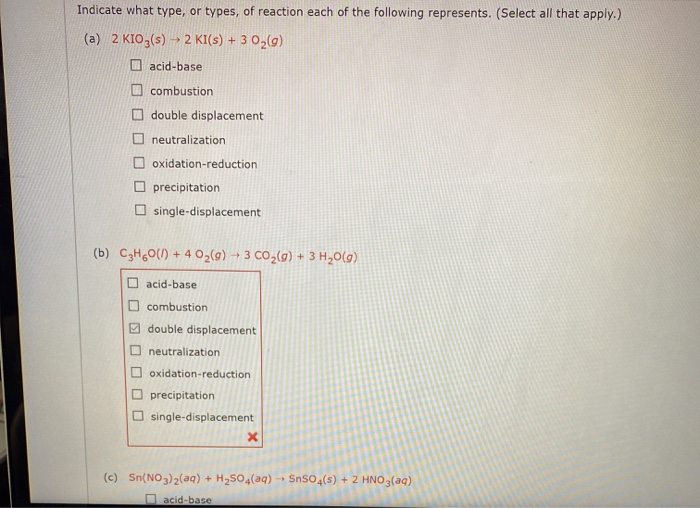Indicate what type, or types, of reaction each of the following represents. (Select all that apply.) (a) 2 KIO3(s) – 2 KI(s) + 3 0,() acid-base combustion double displacement neutralization oxidation-reduction precipitation single-displacement (b) C3H60() + 4 O2(g) + 3 CO2(g) + 3 H2009) acid-base combustion double displacement neutralization oxidation-reduction precipitation single-displacement (c) Sn(NO3)2(aq) + H2SO4(aq) - SnSO4(s) + 2 HNO3(aq) acid-base (c) Sn(NO3)2(aq) + H2SO4(aq) + SnSO4(s) + 2 HNO3(aq) acid-base combustion double displacement neutralization oxidation-reduction precipitation single-displacement (d)...

• ### Write the chemical equation for each reaction described below. Make certain it is balanced, then classify...

Write the chemical equation for each reaction described below. Make certain it is balanced, then classify the reaction as Combination, Decomposition, Single-Replacement, Double-Replacement-Neutralization, Double-Replacement -precipitation, or Combustion. Water is driven from copper sulfate pentahydrate with heat.

• ### ​1. Write a balanced equation for the reaction of HCN in water. Identify the acid, base, conjugate acid and conjugate base.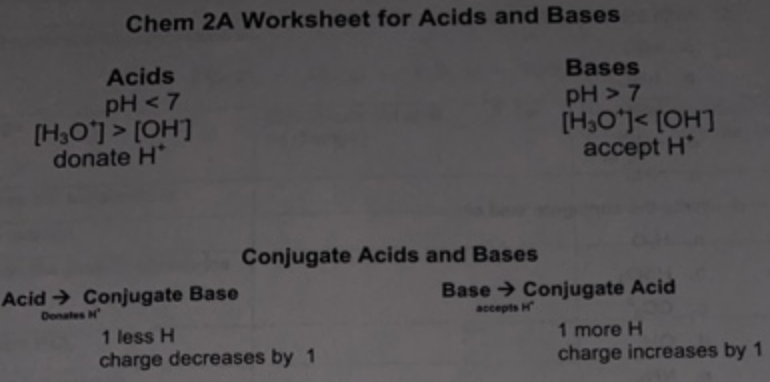1. Write a balanced equation for the reaction of HCN in water. Identify the acid, base, conjugate acid and conjugate base.2. Write a balanced equation for the reaction of NH3 in water. Identify the acid, base, conjugate acid and conjugate base. 3. Write a balanced equation for the reaction of HPO42- in water (where HPO42- is acting as the acid). Identify the acid, base, conjugate acid and conjugate base.4. Write a balanced equation for the reaction of HPO42- 2 in...

• ### Write an equation for the reaction of each of the following with water a) HNO_3 b)...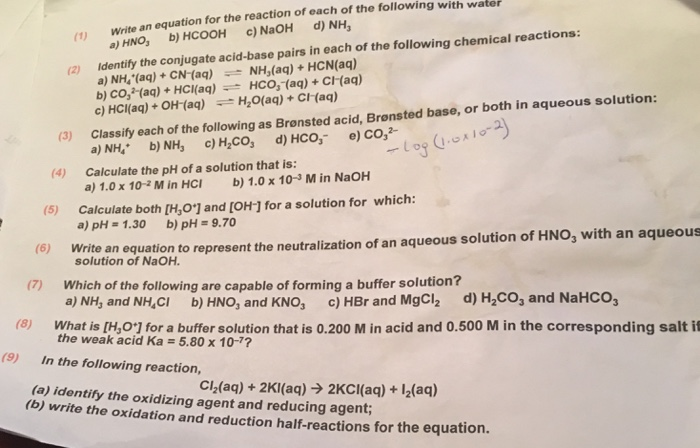Write an equation for the reaction of each of the following with water a) HNO_3 b) HCOOH c) NaOH d) NH_3 (2) Identify the conjugate acid-base pairs in each of the following chemical reactions: a) NH_4^+(aq) + CN^-(aq) NH_3(aq) + HCN(aq) b) CO_3^2-(aq) + HCI(aq) HCO_3^-(aq) + CI^_(aq) c) HCI(aq) + OH^-(aq) H_2O(aq) + CI^-(aq) (3) Classify each of the following as Bronsted acid, Bronsted base, or both in aqueous solution: a) NH_4^+ b) NH_3 c) H_2CO_3 d) HCO_3^- e)...

• ### Write a balanced chemical equation for each reaction. If no reaction occurred, write "N.R."): Magnesium and...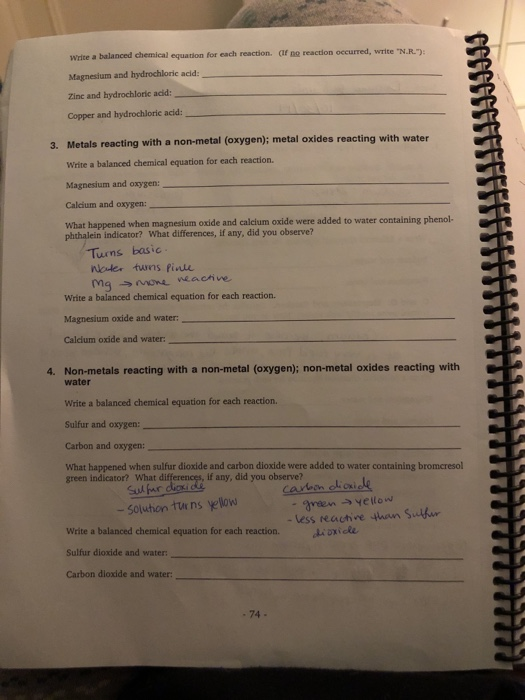Write a balanced chemical equation for each reaction. If no reaction occurred, write "N.R."): Magnesium and hydrochloric acid: Zine and hydrochloric acid: Copper and hydrochloric acid: 3. Metals reacting with a non-metal (oxygen); metal oxides reacting with water Write a balanced chemical equation for each reaction. Magnesium and oxygen: Calcium and oxygen What happened when magnesium oxide and calcium oxide were added to water containing phenol phthalein indicator? What differences, if any, did you observe? Turns basic Water turns Pince...